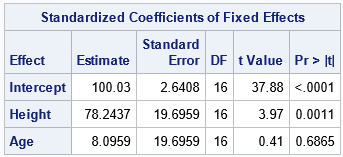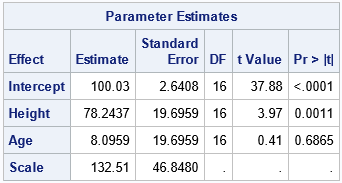I previously wrote about how to understand standardized regression coefficients in PROC REG in SAS. You can obtain the standardized estimates by using the STDB option on the MODEL statement in PROC REG. Several readers have written to ask whether I could write a similar article about the STDCOEFF option on the MODEL statement in PROC GLIMMIX. One reader wrote:

Dear Dr. Wicklin: I get different results when I request standardized regression coefficients from PROC REG and from PROC GLMMIX. I don't understand. Isn't the STB option in PROC REG equivalent to the STDCOEF option in PROC GLIMMIX (for DIST=NORMAL LINK=IDENTITY)? Can you explain what STDCOEF does?

The two options are not equivalent because the procedures use two different methods to standardize the regression coefficients. This article explains the STDCOEF option in PROC GLIMMIX and how it differs from the STDB option in PROC REG.

### How to interpret standardized regression coefficients?

Briefly, researchers look at standardized regression coefficients when the explanatory variables are measured in different units and on different scales. If you do not standardize the data, then a regression coefficient estimates the change in the response variable when you make a unit change in the explanatory variable, holding the other variables constant. As explained in my previous article, the STDB option in PROC REG enables you to interpret the regression in terms of standard deviations. For the standardized coefficients in PROC REG, you can interpret the regression coefficient as the number of standard deviations that the response will change for one "standard deviation" of change in the explanatory variable, holding the other variables constant.

The standardized coefficients in PROC REG have an alternative interpretation: They are the regression estimates that you obtain if you standardize all variables (explanatory AND response) by using the usual standardization formula. The usual standardization is Z* = (Z - mean(Z))/std(Z), where mean(Z) is the sample mean and std(Z) is the sample standard deviation.

If you adopt the second interpretation, then you quickly realize that there are many ways to produce standardize regression coefficients. If you standardize the explanatory variables by any method, you immediately obtain the corresponding set of standardized regression coefficients.

There is no reason why you must include the response variable among the set of variables that you standardize. You can compare the effect of explanatory variables that have different scales without standardizing the response variable (J. Bring, 1994, "How to Standardize Regression Coefficients", Eqn 2.5).

### Other standardizations

The STDCOEF option in PROC GLMIMMIX produces standardized regression coefficients that are different from PROC REG because it uses a different standardization of the variables. First, PROC GLIMMIX does not standardize the response variable. This makes sense for a procedure that supports many different distributions of response variables, such as binary, multinomial, Poisson, and more. Second, instead of dividing each explanatory variable by its standard deviation, PROC GLMIMMIX divides by the square root of the corrected sum of squares (CSS): sqrt(Σi (xi - m)2), where m is the sample mean.

Notice that the CSS is almost the same as the standard deviation, but it lacks the divisor sqrt(N-1), where N is the number of nonmissing observations. In ANOVA computations, the CSS is sometimes called the total sum of squares (TSS). In regression, it is called the residual sum of squares (RSS). Whatever you call it, it represents the deviation of the data from the mean, in squared units. Taking the square root gives a statistic that represents the total deviation of the data from the mean in the original units. The documentation for PROC GLIMMIX includes this formula and states "If you specify the STDCOEF option, fixed-effects parameter estimates and their standard errors are reported in terms of the standardized (scaled and centered) coefficients in addition to the usual results in noncentered form."

That, then, explains the difference between the standardized regression estimates in PROC REG and the estimates in PROC GLIMMIX. The procedures use different standardizations, and PROC REG also standardizes the response variable whereas PROC GLIMMIX does not.

The denominator (sqrt(CSS)), measures the spread of the data much like the standard deviation does, but it is a "total spread" instead of an "average spread." Interpreting a standardized coefficient in PROC GLIMMIX is not very intuitive. You could say that the coefficient estimates the change in the response variable when you make a change of s units in the explanatory variable (holding the other variables constant), where s is a measure of the total spread of the data. Although that sentence is long and wordy, remember that the purpose of the standardized coefficients is to enable you to compare the relative effect of different explanatory variables that are measured on different scales. The PROC GLMMIX standardization accomplishes that purpose.

### A SAS program to compute the standardized regression coefficients

In my previous article, I showed a SAS program that produces the same standardized coefficients as the STDB option in PROC REG. The program is straightforward: Create a new data set that contains the standardized variables and run an ordinary regression with those variables. You can do the same thing to reproduce the STDCOEFF option in PROC GLIMMIX. For simplicity, I will consider a model that has only continuous variables, but to handle classification variables you can generate a design matrix for the explanatory variables and standardize the columns of the design matrix.

Let's use the same example data as in the previous article, which is the Sashelp.Class data set. It contains only 19 observations and has three continuous variables. Let's use Weight as the response variable and Height and Age as the explanatory variables. The following statements use PROC GLIMMIX to fit a fixed-effects model to the data. The STDCOEF option requests standardized regression estimates, which are shown below:

```/* run PROC GLIMMIX on the original data; use STDCOEF option */ proc glimmix data=Sashelp.Class noitprint; model Weight = Height Age / ddfm=kr s STDCOEF; /* request standardization of estimates */ ods select StandardizedCoefficients; run;```According to the discussion in the previous section, you can obtain these same estimates by creating a new data set that contains centered and scaled versions of the explanatory variables. The variables are centered by using their sample means. The variables are scaled by using the square root of their CSS. The mean and CSS statistics are available from PROC MEANS, so you can write these statistics to a SAS data set and use the METHOD=IN option in PROC STDIZE to standardize the variables, as shown in a previous article. For simplicity, I have replaced the standardization code by a macro call so that the main idea is apparent:

```/* Standardize 2 variables (Height and Age) in the Sashelp.Class data. Center by the mean; Scale by the sqrt(CSS). Write the standardized variables to the Want data set. */ %StdizeMeanSqrtCSS(Height Age, 2, Sashelp.Class, Want); /* encapsulates the standardization */   /* run PROC GLIMMIX on the standardized data */ proc glimmix data=Want noitprint; model Weight = Height Age / ddfm=kr s; ods select ParameterEstimates; run;```Voila! As expected, you get the same estimates by using the standardized data as you get when using the original data and the STDCOEF option. You can download the complete SAS program, which includes the details of the standardization.

### Summary

This article explains the STDCOEF option in PROC GLIMMIX and how it differs from the STDB option in PROC REG. PROC REG standardizes both the explanatory and response variables. It uses the formula (X - mean(X))/std(X) to standardize variables. In contrast, PROC GLIMMIX does not standardize the response variable. The explanatory variables are standardized by using the formula (X - mean(X))/sqrt(CSS(X)).

The post Standardized regression coefficients in PROC GLIMMIX appeared first on The DO Loop.